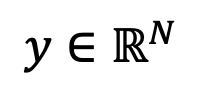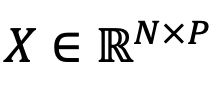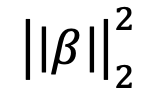# Glossary

## Ridge Regression

##### What is Ridge Regression?

Ridge regression, or Tikhonov regularization, is an extension of ordinary least squares (linear) regression with an additional l2-penalty term (or ridge constraint) to regularize the regression coefficients. Machine learning models that leverage ridge regression identify the optimal set of regression coefficients as followswhereis the dependent/target variable whose value the model is trying to predict using N samples of training data,, and P features. The “shrinkage parameter” or “regularization coefficient”, λ, controls the l2 penalty term on the regression coefficients,. Increasing λ forces the regression coefficients in the AI model to become smaller. If λ = 0, the formulation is equivalent to ordinary least squares regression.

##### Why is Ridge Regression Important?

Ridge regression adds the l2-penalty term to ensure that the linear regression coefficients do not explode (or become very large). It reduces variance, producing more consistent results on unseen datasets. It also helps deal with multicollinearity, which happens when the P features in X are linearly dependent.

##### How C3 AI Helps Organizations Use Ridge Regression

Ridge regression is supported as a machine learning technique in the C3 AI Platform. It is infrequently used in practice because data scientists favor more generally applicable, non-linear regression techniques, such as random forests, which also serve to reduce variance in unseen datasets. However, it works well when there is a strong linear relationship between the target variable and the features. It has been used in a C3 AI Reliability proof of technology for a customer that wanted to predict shell temperatures in industrial heat exchangers using fouling factors as features.